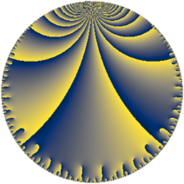Properties

 Label 56.7.oLevel $56$ Weight $7$ Character orbit 56.o Rep. character $\chi_{56}(17,\cdot)$ Character field $\Q(\zeta_{6})$ Dimension $24$ Newform subspaces $1$ Sturm bound $56$ Trace bound $0$

Related objects

Defining parameters

 Level: $$N$$ $$=$$ $$56 = 2^{3} \cdot 7$$ Weight: $$k$$ $$=$$ $$7$$ Character orbit: $$[\chi]$$ $$=$$ 56.o (of order $$6$$ and degree $$2$$) Character conductor: $$\operatorname{cond}(\chi)$$ $$=$$ $$7$$ Character field: $$\Q(\zeta_{6})$$ Newform subspaces: $$1$$ Sturm bound: $$56$$ Trace bound: $$0$$

Dimensions

The following table gives the dimensions of various subspaces of $$M_{7}(56, [\chi])$$.

Total New Old
Modular forms 104 24 80
Cusp forms 88 24 64
Eisenstein series 16 0 16

Trace form

 $$24 q + 564 q^{7} + 2300 q^{9} + O(q^{10})$$ $$24 q + 564 q^{7} + 2300 q^{9} + 812 q^{11} - 11752 q^{15} - 840 q^{17} - 2268 q^{19} + 68 q^{21} + 1392 q^{23} + 28104 q^{25} + 51464 q^{29} + 139860 q^{31} - 124572 q^{33} - 87564 q^{35} - 22548 q^{37} + 45476 q^{39} - 221472 q^{43} + 15204 q^{45} - 124740 q^{47} - 83184 q^{49} + 224772 q^{51} + 143692 q^{53} + 227176 q^{57} - 378840 q^{59} + 345492 q^{61} - 166556 q^{63} + 351380 q^{65} - 744 q^{67} + 1821328 q^{71} - 918036 q^{73} - 1826496 q^{75} - 826704 q^{77} - 756960 q^{79} - 188640 q^{81} - 1589448 q^{85} + 1642452 q^{87} + 1809444 q^{89} + 3269160 q^{91} + 1843780 q^{93} - 1423224 q^{95} + 68552 q^{99} + O(q^{100})$$

Decomposition of $$S_{7}^{\mathrm{new}}(56, [\chi])$$ into newform subspaces

Label Dim. $$A$$ Field CM Traces $q$-expansion
$a_{2}$ $a_{3}$ $a_{5}$ $a_{7}$
56.7.o.a $24$ $12.883$ None $$0$$ $$0$$ $$0$$ $$564$$

Decomposition of $$S_{7}^{\mathrm{old}}(56, [\chi])$$ into lower level spaces

$$S_{7}^{\mathrm{old}}(56, [\chi]) \cong$$ $$S_{7}^{\mathrm{new}}(7, [\chi])$$$$^{\oplus 4}$$$$\oplus$$$$S_{7}^{\mathrm{new}}(14, [\chi])$$$$^{\oplus 3}$$$$\oplus$$$$S_{7}^{\mathrm{new}}(28, [\chi])$$$$^{\oplus 2}$$